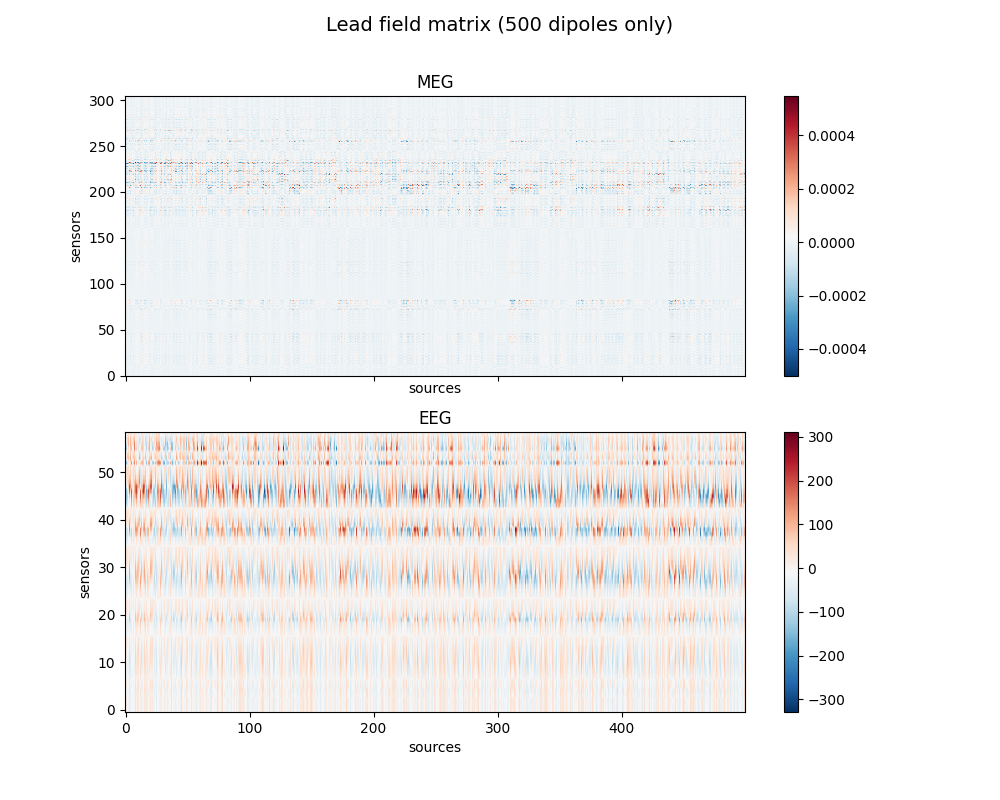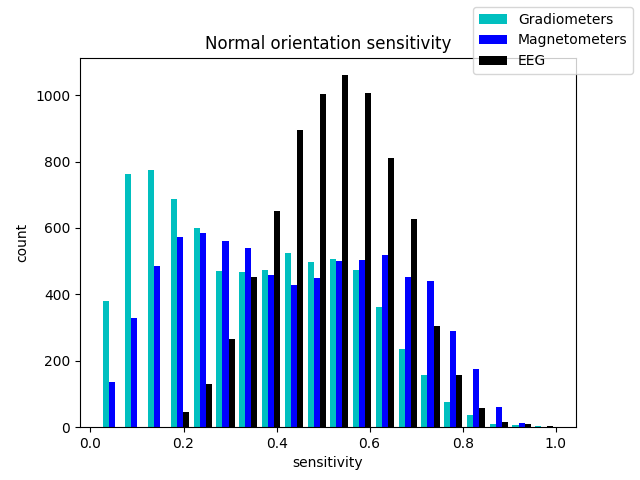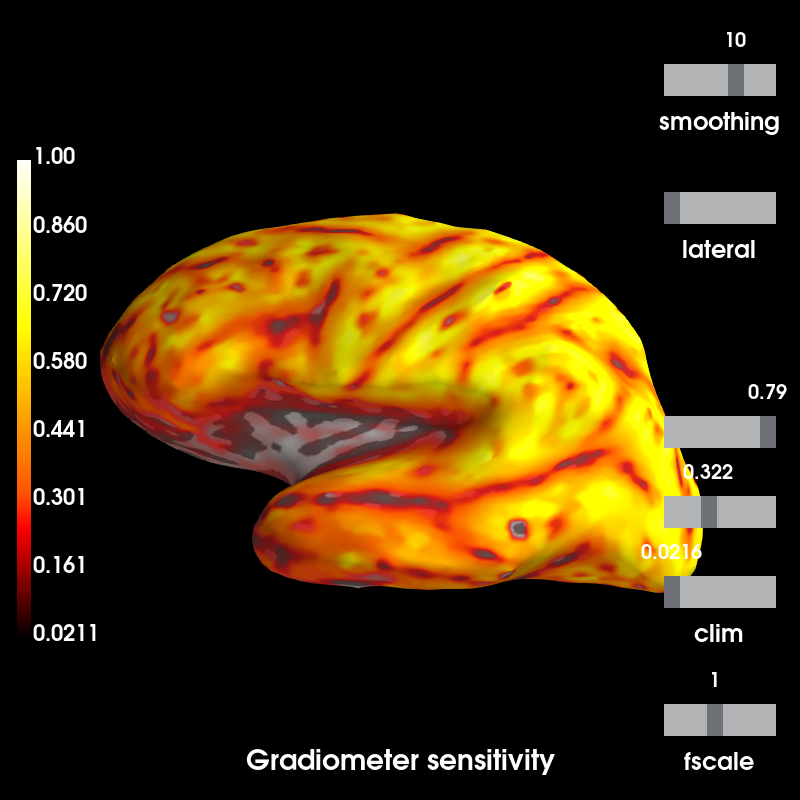# Display sensitivity maps for EEG and MEG sensors¶

Sensitivity maps can be produced from forward operators that indicate how well different sensor types will be able to detect neural currents from different regions of the brain.

To get started with forward modeling see Head model and forward computation.

# Author: Eric Larson <larson.eric.d@gmail.com>
#

import mne
from mne.datasets import sample
import matplotlib.pyplot as plt

print(__doc__)

data_path = sample.data_path()

raw_fname = data_path + '/MEG/sample/sample_audvis_raw.fif'
fwd_fname = data_path + '/MEG/sample/sample_audvis-meg-eeg-oct-6-fwd.fif'

subjects_dir = data_path + '/subjects'

# Read the forward solutions with surface orientation
mne.convert_forward_solution(fwd, surf_ori=True, copy=False)


Out:

Reading forward solution from /home/circleci/mne_data/MNE-sample-data/MEG/sample/sample_audvis-meg-eeg-oct-6-fwd.fif...
Computing patch statistics...
[done]
Computing patch statistics...
[done]
Desired named matrix (kind = 3523) not available
Read MEG forward solution (7498 sources, 306 channels, free orientations)
Desired named matrix (kind = 3523) not available
Read EEG forward solution (7498 sources, 60 channels, free orientations)
MEG and EEG forward solutions combined
Source spaces transformed to the forward solution coordinate frame
Average patch normals will be employed in the rotation to the local surface coordinates....
Converting to surface-based source orientations...
[done]
Leadfield size : 366 x 22494


Compute sensitivity maps

grad_map = mne.sensitivity_map(fwd, ch_type='grad', mode='fixed')
mag_map = mne.sensitivity_map(fwd, ch_type='mag', mode='fixed')
eeg_map = mne.sensitivity_map(fwd, ch_type='eeg', mode='fixed')


Out:

    204 out of 366 channels remain after picking
102 out of 366 channels remain after picking
60 out of 366 channels remain after picking


Show gain matrix a.k.a. leadfield matrix with sensitivity map

picks_meg = mne.pick_types(fwd['info'], meg=True, eeg=False)
picks_eeg = mne.pick_types(fwd['info'], meg=False, eeg=True)

fig, axes = plt.subplots(2, 1, figsize=(10, 8), sharex=True)
fig.suptitle('Lead field matrix (500 dipoles only)', fontsize=14)
for ax, picks, ch_type in zip(axes, [picks_meg, picks_eeg], ['meg', 'eeg']):
im = ax.imshow(leadfield[picks, :500], origin='lower', aspect='auto',
cmap='RdBu_r')
ax.set_title(ch_type.upper())
ax.set_xlabel('sources')
ax.set_ylabel('sensors')
fig.colorbar(im, ax=ax)

fig_2, ax = plt.subplots()
color=['c', 'b', 'k'])
fig_2.legend()
ax.set(title='Normal orientation sensitivity',
xlabel='sensitivity', ylabel='count')

clim=dict(lims=[0, 50, 100]))

••Out:

Using control points [0.02108201 0.32186553 1.        ]


Total running time of the script: ( 0 minutes 8.402 seconds)

Estimated memory usage: 77 MB

Gallery generated by Sphinx-Gallery